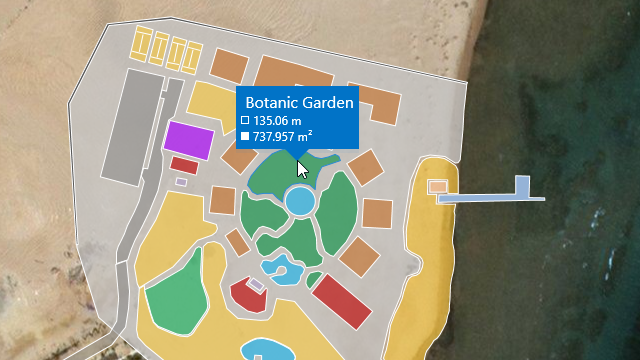21.2 (EAP/Beta)
21.1
20.2
20.1
19.2
19.1
18.2
The page you are viewing does not exist in version 18.2. This link will take you to the root page.
18.1
The page you are viewing does not exist in version 18.1. This link will take you to the root page.
17.2
The page you are viewing does not exist in version 17.2. This link will take you to the root page.
You are viewing help content for pre-release software. This document and the features it describes are subject to change. Switch to the current version.

# GeoUtils Class

Cartography measurement API for maps with a geographical coordinate system.

Namespace: DevExpress.Xpf.Map

Assembly: DevExpress.Xpf.Map.v21.2.dll

## Declaration

``public static class GeoUtils``

## Remarks

The `GeoUtils` class contains methods that allow you to calculate geometric values (for example, a shape perimeter or area) based on geographical coordinates.The following list contains the main `GeoUtils` methods:

• CalculateArea(MapShape shape) computes a map shape’s total area, in square meters.

``````private void MapControl_SelectionChanged(object sender, MapItemSelectionChangedEventArgs e) {
MapShape mapShape = vectorLayer.SelectedItems as MapShape;
double areaByShape = GeoUtils.CalculateArea(mapShape) / 1000000;
label.Content = \$"Shape Area: {areaByShape:F2} km²";
}
``````
• CalculateArea(IList<GeoPoint> points) calculates an area of the region given by geographical points, in square meters.

``````double areaByPoints = GeoUtils.CalculateArea(new List<GeoPoint> {
new GeoPoint(41, -109),
new GeoPoint(41, -102),
new GeoPoint(37, -102),
new GeoPoint(37, -109)
});
``````
• CalculateBearing(GeoPoint p1, GeoPoint p2) computes an angle between the Geodetic North direction and a line given by two points, clockwise in degrees.

``````double bearing = GeoUtils.CalculateBearing(new GeoPoint(36.1, -115.1), new GeoPoint(35.9, -115.9));
``````
• CalculateCenter(GeoPoint p1, GeoPoint p2) finds a center point between two geographical points.

``````GeoPoint centerPoint = GeoUtils.CalculateCenter(
new GeoPoint(35.286, -111.1724),
new GeoPoint(38.3802, -110.9307));
``````
• CalculateDistance(GeoPoint p1, GeoPoint p2) determines the distance between two geographical points in meters. The ellipsoidal model of the Earth is used in the calculations of the distance.

``````double distance = GeoUtils.CalculateDistance(new GeoPoint(36.1, -115.1), new GeoPoint(34, -118.2));
``````
• CalculateDistance(GeoPoint p1, GeoPoint p2, Boolean ignoreEllipsoidalEffects) determines the distance between two geographical points in meters. This `CalculateDistance` method overload allows you to specify whether to use the ellipsoidal-surface formula or spherical-surface formula to calculate the distance.

``````using DevExpress.Xpf.Map;

private void OnButtonClick(object sender, RoutedEventArgs e) {
double distance = GeoUtils.CalculateDistance(new GeoPoint(-5.93107, -35.112723),
new GeoPoint(4.253438, 5.47072), true);
}
``````
• CalculateDestinationPoint(GeoPoint startPoint, Double distance, Double bearing) calculates a geographical point based on the specified start point, distance, and bearing. The distance is measured along a geodesic line.

``````GeoPoint startPoint = new GeoPoint(53.1914, 001.4347);
GeoPoint destinationPoint = GeoUtils.CalculateDestinationPoint(startPoint, 17910000, 276.0117);
``````
• CalculateLength(IList<GeoPoint> points) calculates the polyline length, in meters.

``````double lineLength = GeoUtils.CalculateLength(new List<GeoPoint> {
new GeoPoint(35.286, -111.1724),
new GeoPoint(38.3802, -110.9307),
new GeoPoint(36.9365, -102.493)
});
``````
• CalculateStrokeLength(MapShape shape) returns the map shape’s stroke length, in meters.

``````private void MapControl_SelectionChanged(object sender, MapItemSelectionChangedEventArgs e) {
MapShape mapShape = vectorLayer.SelectedItems as MapShape;
double strokeLength = GeoUtils.CalculateStrokeLength(mapShape);
label.Content = \$"StokeLength: {strokeLength / 1000:F2} km";
}
``````

Object
GeoUtils